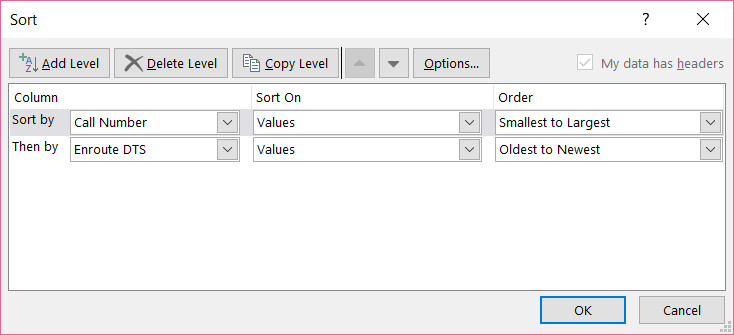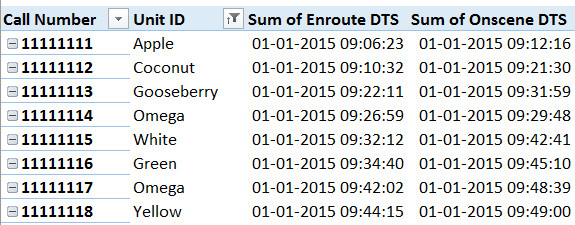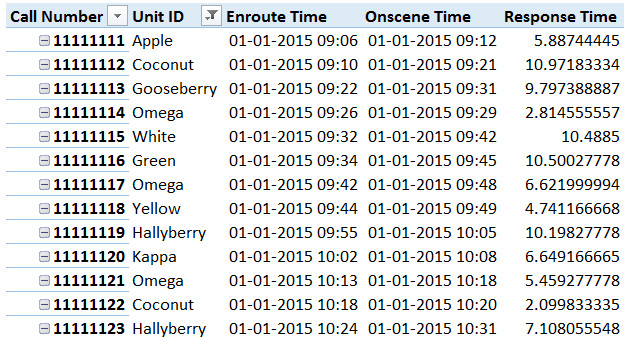# Analyzing 300,000 calls for help [case study]

Over the weekend, I got an email from Mr. E, one of my students. Mr. E works at a police department in California and as part of his work, he was looking at calls received by police. Whenever police get a call for help, multiple teams can respond to the call and go to the location. All of these dispatches are recorded. So a single call can have several such dispatches. And Mr. E wanted to findout which team responded the first. The problem?

Finding the first responded team is tricky.

Today let’s take up this problem as a case study and understand various methods to solve it.  We are going to learn about writing better lookups, pivot tables, power pivot and optimization. Put on your helmets, cause this is going to be mind blowingly awesome.

### A little background

We are re-opening enrollments in to the 50 ways to analyze data program in two weeks (on 7th of September, Wednesday). I want to share the process, techniques and visualizations you can use to analyze any business data with a case study. In this series of articles, let’s analyze fairly complex and large data set to derive insights.

Please join our Analytics Email Newsletter to get all three parts of this series and information about 50 Ways to Analyze Data program.

## Let’s look at the data

Since the original data shared by Mr. E is confidential, I made up some random call data in Excel. Here is a sample.The calls data is maintained in an Excel table, named calls.

We have 288,625 rows in this table. Let’s examine the columns in detail.

• Call number – a unique identifier for the call
• Unit id – Name of unit responding to call
• Call time – Time stamp when we got the call. This is repeated for all rows with same call number
• Enroute DTS – Time stamp when unit left to attend call
• Onscene DTS – Time stamp when unit reached scene

## The problem – How soon do we help?

We need to answer the question – how soon are we helping the callers?

This can be answered in a variety of ways.

• Calculate the earliest response time for each call by,
• difference between call time and earliest Onscene DTS
• difference between earliest Enroute DTS (ie first unit to respond) and corresponding Onscene DTS
• Analyzing the response times
• finding key statistics – ie average, median, quartiles and outliers
• Slicing the stats by month, call region (data not available) or team

Mr. E’s email was about how to calculate the earliest Enroute DTS and corresponding Onscene DTS values.

In this article, we will explore ways to calculate these values using various Excel techniques. In the next article, we will analyze response times by various dimensions to understand the trends better.

## Calculating earliest enroute time – so many ways to get there

We can use a variety of techniques to calculate the earliest enroute time.

• MIN IF formula to find the minimum time among all for a particular call number
• VLOOKUP exact match on sorted data table
• INDEX + approximate MATCH on sorted data table
• Pivot tables
• Power Pivot

Let’s review each of these techniques and see which ones are easy, fast and accurate (ie awesome)

### MIN IF formula

This is where Mr. E got stuck and emailed me. He was implementing an array formula to fetch the earliest enroute time using MIN and IF formulas.

Assuming a call number is listed in cell H4, we can fetch earliest enroute time using below MINIF formula.

`=MIN(IF(calls[Call Number]=H4,calls[Enroute DTS]))`

While this formula is technically correct, Mr. E faced a curious problem. Not all units chose to attend a call even after taking it. This could be because they chose to go on another call or realized that another team is already on the way. So quite a few items in [Enroute DTS] are blanks ie 0. Now, because we are using MIN formula, it fetches 0 as the earliest Enroute DTS, which is clearly a wrong answer.

We can add an additional check to handle blank [Enroute DTS] entries.

`=MIN(IF((calls[Call Number]=H4)*(calls[Enroute DTS]>0),calls[Enroute DTS]))`

Now this works.

Verdict on MINIF formula approach

While MINIF formula is a simple & elegant solution to this problem, it is not scalable. Mr. E had close to 300,000 calls spread among 400,000 rows in the calls table. This means when you want to calculate earliest Enroute DTS for all the 300,000 calls using MINIF, you can take a very looooong coffee break.

### VLOOKUP approach

We can use VLOOKUP to return first occurrence of Enroute DTS for a given call number. We needed earliest value of Enroute DTS, not the first value.

Problem?

Well, what if we sort the calls table by call number and Enroute DTS?Now, VLOOKUP should fetch earliest Enroute DTS.

`=VLOOKUP(M4,calls,4,FALSE)`

should fetch the earliest Enroute DTS for a call number in M4.

Verdict on VLOOKUP approach

While VLOOKUP is easier than MINIF and gives us the answer we want, it too is not scalable. On my sample data of 300,000 rows, the VLOOKUP approach took more than 2 minutes. On a production data set, this approach could mean disaster.

### INDEX + approximate MATCH approach

Why bother with exact lookups when an approximate one would do? That is logic behind this approach.

Assuming the call numbers are listed in M4 onwards,

• We find the first call’s earliest Enroute DTS using regular VLOOKUP method as mentioned above.
• From second call number onwards, we use an approximate MATCH method like below

`=INDEX(calls[Enroute DTS],MATCH(M4,calls[Call Number],1)+1)`

Notice that we refer M4 to fetch earliest Enroute DTS for M5.

Related: INDEX formulaINDEX + MATCH formulas

Verdict on INDEX + MATCH approach

This approach is significantly faster than VLOOKUP or MINIF solution. On test runs with same dataset, this approach took just a fraction of second. That is roughly 100 times improvement in speed.

If you must use a formula approach, use this.

### Pivot Tables

Pivot tables are a great way to answer questions like these. You can create a quick pivot to find earliest Enroute DTS for all calls by,• Insert a pivot from the calls table
• Add call number & unit ID to row labels area
• Add Enroute DTS and Onscene DTS to values column
• Change calculation for these value fields to SUM (as by default dates are COUNTed)
• Sort the pivot by Enroute DTS – Oldest to newest
• Change the report layout to tabular view
• Go to value filter for Unit ID and filter by bottom 1 value by Enroute DTS
• Now you get a sparkly clean pivot with what we need.Verdict on Pivot Table approach

This is the quickest of them all. Very simple and scalable upto a million rows (although when you have so many rows, Pivot calculations can be slow). I recommend that you use Pivot Table approach whenever you are solving problems like this.

### Power Pivot

While pivot table approach gives us the answer we want in a quick and efficient fashion, it has few limitations.

• Pivot tables cant work when you have more than a million calls
• Doing further analysis by slicing and dicing the data on various dimensions can be hard

This is where Power Pivot truly shines.

Related: Introduction to Excel Power Pivot.

Let’s build a Power Pivot model around the problem.

We load calls data in to PP as linked table (but in a production environment, you can directly hookup to database containing the data, thus making your workbook smaller)

Now create below measures.

Enroute Time:= min(calls[Enroute DTS])

Onscene Time:= min(calls[Onscene DTS])

Response Time:= [Onscene Time] – [Enroute Time]

Additionally, we can also create Earliest Enroute Time measure as,

Earliest Enroute Time:= calculate ([Enroute Time], all(calls[Unit ID]))

Now, add the first 3 measures to a pivot table. Place call number & unit id in row labels area. Set up tabular layout for the pivot and viola, our answer is ready.So there you go.

Verdict on Power Pivot approach:

If you have Power Pivot on your Excel, use this method all the time. This allows you lots of flexibility. You can also build additional measures to analyze the data. We will see some of these ideas in next part of this article.

Click here to download sample workbook containing all these solutions. Play with various formulas and measures to learn more. Beware, some of the formulas can take lot of time to re-calculate.

## What next?

The purpose of any analysis is to uncover patterns, information and decision pointers buried in data. So far, we just scratched the surface of this data set. In the next installment, let’s dig deeper and see meaningful trends.

### A testimonial from Mr. E …

After helping Mr. E, he sent me below email. This is one of the reasons why I run Chandoo.org. Everytime I read a testimonial like this, I get a big bright smile on my face.

I just wanted to say thank you very much. Although some of my columns are still calculating (holy smokes it takes a long time), the ones I’ve applied this solution to are working brilliantly.

You’ve helped me solve a very big problem for the xxxx county sheriff’s office in California; serving some 400k people. I thought that might be a nice feather to put in your hat.

Thank you E for emailing an interesting problem.